## GATE 2007-IT

 Question 1

Suppose there are two coins. The first coin gives heads with probability 5/8 when tossed, while the second coin gives heads with probability 1/4. One of the two coins is picked up at random with equal probability and tossed. What is the probability of obtaining heads ?

 A 7/8 B 1/2 C 7/16 D 5/32
Engineering-Mathematics       Probability
Question 1 Explanation:
Each coin has equal probability to pick i.e., 1/2.
The probability of obtaining heads
= (1/2)(5/8) + (1/2)(1/4)
= (5/16) + (1/8)
= 7/16
 Question 2

Let A be theWhat is the maximum value of xTAx where the maximum is taken over all x that are the unit eigenvectors of A?

 A 5 B 5+√5/2 C 3 D 5-√5/2
Engineering-Mathematics       Linear-Algebra
Question 2 Explanation: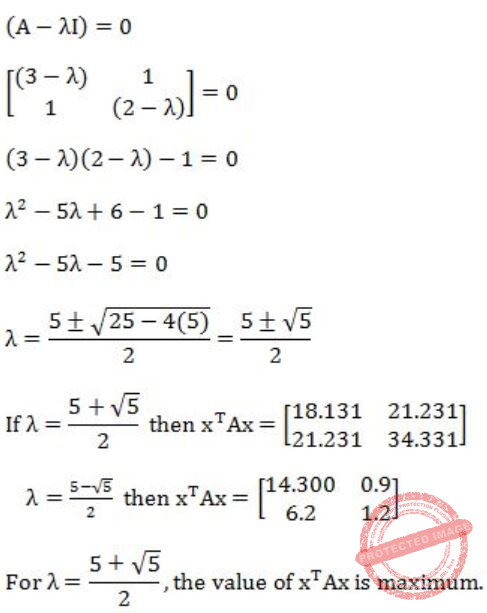Question 3

Consider a weighted undirected graph with positive edge weights and let uv be an edge in the graph. It is known that the shortest path from the source vertex s to u has weight 53 and the shortest path from s to v has weight 65. Which one of the following statements is always true?

 A weight (u, v) < 12 B weight (u, v) ≤ 12 C weight (u, v) > 12 D weight (u, v) ≥ 12
Algorithms        Shortest Path
Question 3 Explanation:
Correct answer will be,
weight (u,v) ≥ 12
Lets check other options,
A) If the weight (u,v) < 12
Minimum weight of (s,v)
= weight of (s,v) + weight of (u,v)
= 53 + (<12)
= less than 65, which is not possible because shortest path from s to v has weight 65.
Similarly, we can check for option (B) and (C).
 Question 4

In the Spiral model of software development, the primary determinant in selecting activities in each iteration is

 A Iteration size B Cost C Adopted process such as Rational Unified Process or Extreme Programming D Risk
Software-Engineering       Software-process-models
Question 4 Explanation:
Note: Out of syllabus.
 Question 5

Which of the following systems is a most likely candidate example of a pipe and filter architecture ?

 A Expert system B DB repository C Aircraft flight controller D Signal processing
Signal-processing       pipe-and-filter-architecture
Question 5 Explanation:
Note: Out of syllabus.
 Question 6

A processor takes 12 cycles to complete an instruction I. The corresponding pipelined processor uses 6 stages with the execution times of 3, 2, 5, 4, 6 and 2 cycles respectively. What is the asymptotic speedup assuming that a very large number of instructions are to be executed?

 A 1.83 B 2 C 3 D 6
Computer-Organization       Pipelining
Question 6 Explanation:
Let there be n instructions.
For a non-pipelined processor each instruction takes 12 cycles.
So for n instructions total execution time be 12 × n = 12n
For a pipelined processor each instruction takes
max (3, 2, 5, 4, 6, 2) = 6
So for n instructions total execution time be,
(1×6 + (n-1) × 1) × 6
= (6 + n - 1) × 6
= (5 + n) × 6
= 30 + 6n
∴ Speedup = time without pipeline/time with pipeline = 12n/30+6n
So, if n is very large,Question 7

Which of the following input sequences for a cross-coupled R-S flip-flop realized with two NAND gates may lead to an oscillation?

 A 11, 00 B 01, 10 C 10, 01 D 00, 11
Digital-Logic-Design       Sequential-Circuits
Question 7 Explanation:
RS slip-flop using NAND gates.
So, 00 input cause indeterminate state which may lead to oscillation.
 Question 8

The following circuit implements a two-input AND gate using two 2-1 multiplexers. What are the values of X1, X2, X3 ?A X1 = b, X2 = 0, X3 = a B X1 = b, X2 = 1, X3 = b C X1 = a, X2 = b, X3 = 1 D X1 = a, X2 = 0, X3 = b
Digital-Logic-Design       Multiplexer
Question 8 Explanation:
F = (bX1' + aX1)X3 + X2X3'
If we put
X1 = b
X2 = 0
X3 = a
Then we get,
F = ab
 Question 9

Consider an ambiguous grammar G and its disambiguated version D. Let the language recognized by the two grammars be denoted by L(G) and L(D) respectively. Which one of the following is true ?

 A L (D) ⊂ L (G) B L (D) ⊃ L (G) C L (D) = L (G) D L (D) is empty
Compiler-Design       Grammar
Question 9 Explanation:
By changing the corresponding grammar, the language will not be changed.
For example, by converting NFA to DFA language will not be changed.
 Question 10

Processes P1 and P2 use critical_flag in the following routine to achieve mutual exclusion. Assume that critical_flag is initialized to FALSE in the main program.

```        get_exclusive_access ( )
{
if (critical _flag == FALSE) {
critical_flag = TRUE ;
critical_region () ;
critical_flag = FALSE;
}
}```

Consider the following statements. i. It is possible for both P1 and P2 to access critical_region concurrently. ii. This may lead to a deadlock.

Which of the following holds?

 A (i) is false and (ii) is true B Both (i) and (ii) are false C (i) is true and (ii) is false D Both (i) and (ii) are true
Operating-Systems       Process-Synchronization
Question 10 Explanation:
Both the processes run concurrently if they run concurrently till the execution then there is no deadlock.
 Question 11

Let a memory have four free blocks of sizes 4k, 8k, 20k, 2k. These blocks are allocated following the best-fit strategy. The allocation requests are stored in a queue as shown below.The time at which the request for J7 will be completed will be

 A 16 B 19 C 20 D 37
Operating-Systems       Process-Scheduling
Question 11 Explanation:
At t = 0At t = 8At t = 10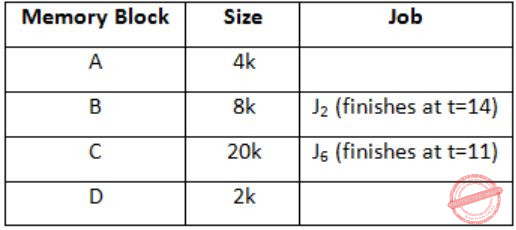At t = 11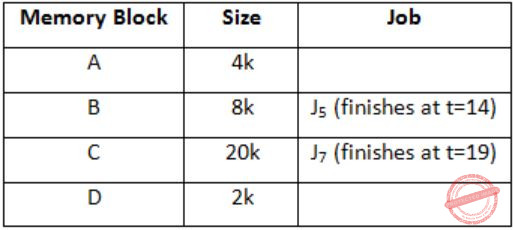J7 can be finished at t = 19.
 Question 12

The address sequence generated by tracing a particular program executing in a pure demand paging system with 100 bytes per page is

` 0100, 0200, 0430, 0499, 0510, 0530, 0560, 0120, 0220, 0240, 0260, 0320, 0410. `
Suppose that the memory can store only one page and if x is the address which causes a page fault then the bytes from addresses x to x + 99 are loaded on to the memory. How many page faults will occur?

 A 0 B 4 C 7 D 8
Question 12 Explanation:
0100 - Page fault, address till 199 in memory
0200 - Page fault, address till 299 in memory
0430 - Page fault, address till 529 in memory
0499 - No page fault
0510 - No page fault
0530 - Page fault, address till 629 in memory
0560 - No page fault.
0120 - Page fault, address till 219 in memory
0220 - Page fault, address till 319 in memory
0240 - No page fault
0260 - No page fault
0320 - Page fault, address till 419 in memory
0410 - No page fault
So, total page faults = 7.
 Question 13

Consider the following statements about the timeout value used in TCP.
(i) The timeout value is set to the RTT (Round Trip Time) measured during TCP connection establishment for the entire duration of the connection.
(ii) Appropriate RTT estimation algorithm is used to set the timeout value of a TCP connection.
(iii) Timeout value is set to twice the propagation delay from the sender to the receiver.
Which of the following choices hold?

 A (i) is false, but (ii) and (iii) are true B (i) and (iii) are false, but (ii) is title C (i) and (ii) are false, but (iii) is true D (i), (ii) and (iii) are false
Computer-Networks       TCP
Question 13 Explanation:
Statement-I: It is False.
The timeout value cannot be fixed for entire duration as it will turn timer to static timer, we need dynamic timer for timeout.
Statement-II: It is True.
Basic algorithm, Jacobson's algorithm, Karl's modification; these three algorithms are to be appropriate to RTT estimation algorithm used to set timeout value dynamically.
Statement-III: It is False.
Because timeout value is set to twice the propagation delay in data link layer where hop to hop distance is known, not in TCP layer.
 Question 14

Consider a TCP connection in a state where there are no outstanding ACKs. The sender sends two segments back to back. The sequence numbers of the first and second segments are 230 and 290 respectively. The first segment was lost, but the second segment was received correctly by the receiver. Let X be the amount of data carried in the first segment (in bytes), and Y be the ACK number sent by the receiver. The values of X and Y (in that order) are

 A 60 and 290 B 230 and 291 C 60 and 231 D 60 and 230
Computer-Networks       TCP
Question 14 Explanation:
In the 1st segment data is from byte number 230 to byte number 289, that is 60 bytes. As 1st segment is lost, so TCP will send ACK for the next-in-order segment receiver is to be expecting. So, it will be for 230.
 Question 15

Consider the following two statements:
(i) A hash function (these are often used for computing digital signatures) is an injective function.
(ii) An encryption technique such as DES performs a permutation on the elements of its input alphabet.
Which one of the following options is valid for the above two statements?

 A Both are false B Statement (i) is true and the other is false C Statement (ii) is true and the other is false D Both are true
Computer-Networks       Network-Security
Question 15 Explanation:
i) Hash function is many to one function. It is not one-one (or) injective.
ii) It uses the P-Box permutation.
Statement-I is false, II is true.
 Question 16

The minimum positive integer p such that 3p modulo 17 = 1 is

 A 5 B 8 C 12 D 16
Engineering-Mathematics       Numerical-Methods
Question 16 Explanation:
According to Fermat's little theorem,
ap-1 mod p = 1
Here, p = 17
So, p-1 = 16 is the answer.
 Question 17

Exponentiation is a heavily used operation in public key cryptography. Which of the following options is the tightest upper bound on the number of multiplications required to compute bn mod m, 0≤b, n≤m?

 A O(log n) B O(√n) C O(n/log n) D O(n)
0       Algorithms
Question 17 Explanation:
In this we need to compute like
C1 = bn/2 × bn/2
C2 = bn/4 × bn/4
C3 = bn/8 × bn/8

Ck = b2 × b2
Recurrence relation T(n) = T(n/2) + O(1)
T(n) = O(log n)
 Question 18

A firewall is to be configured to allow hosts in a private network to freely open TCP connections and send packets on open connections. However, it will only allow external hosts to send packets on existing open TCP connections or connections that are being opened (by internal hosts) but not allow them to open TCP connections to hosts in the private network. To achieve this the minimum capability of the firewall should be that of

 A A combinational circuit B A finite automaton C A pushdown automaton with one stack D A pushdown automaton with two stacks
Computer-Networks       TCP
Question 18 Explanation:
Pushdown automata with two stacks which is the Turing machine.
Turing machine can do everything as the normal computer can do, so firewall can be created by the TM.
 Question 19

Given below are HTML lines,With reference to the HTML lines given above, consider the following statements.
(i) Clicking on the point <80, 75> does not have any effect.
(ii) The web browser can identify the area applicable to the mouse-click within the image and the subsequent action to be taken without additional responses from the web server.
(iii) The dots in the cgi-bin URL will be resolved by the web browser before it is sent to the web server.
(iv) The "fd.html" request when sent to the web server will result in a GET request.
Exactly how many of the statements given above are correct?

 A 0 B 1 C 2 D 3
Question 19 Explanation:
Note: Out of syllabus.
 Question 20

Consider the XML document fragment given below:Consider the XPath expression: *[not (self)::TOC]
What would be the result of the given XPath expression when the current node is Book?

 A The Title and Content elements B The Content and TOC elements C The Title and TOC elements D The Title, Content and TOC elements
Question 20 Explanation:
Note: Out of syllabus.
 Question 21

Which one of these first-order logic formula is valid?

 A ∀x(P(x) ⇒ Q(x)) ⇒ (∀xP(x) ⇒ ∀xQ(x)) B ∃x(P(x) ∨ Q(x)) ⇒ (∃xP(x) ⇒ ∃xQ(x)) C ∃x(P(x) ∧ Q(x)) (∃xP(x) ∧ ∃xQ(x)) D ∀x∃y P(x, y) ⇒ ∃y∀x P(x, y)
Engineering-Mathematics       Propositional-Logic
Question 21 Explanation:
LHS = for every x, if P holds then Q holds
RHS = if P(x) holds for all x, then Q(x) holds for all x
LHS ⇒ RHS (✔)
RHS ⇒ LHS (️×)
 Question 22

The trapezoidal method is used to evaluate the numerical value of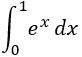. Consider the following values for the step size h.

(i) 10-2
(ii) 10-3
(iii) 10-4
(iv) 10-5

For which of these values of the step size h, is the computed value guaranteed to be correct to seven decimal places. Assume that there are no round-off errors in the computation.

 A (iv) only B (iii) and (iv) only C (ii), (iii) and (iv) only D (i), (ii), (iii) and (iv)
Engineering-Mathematics       Trapezoidal Method
Question 22 Explanation:
Note: Out of syllabus.
 Question 23

A partial order P is defined on the set of natural numbers as follows. Here x/y denotes integer division.
(i) (0, 0) ∊ P.
(ii) (a, b) ∊ P if and only if a % 10 ≤ b % 10 and (a/10, b/10) ∊ P.
Consider the following ordered pairs:
(i) (101, 22)
(ii) (22, 101)
(iii) (145, 265)
(iv) (0, 153)
Which of these ordered pairs of natural numbers are contained in P?

 A (i) and (iii) B (ii) and (iv) C (i) and (iv) D (iii) and (iv)
Engineering-Mathematics       Sets-And-Relation
Question 23 Explanation:
For ordered pair (a, b) to be in P, each digit in starting from unit place must not be larger than the corresponding digit in b.
The given condition can be satisfied by
(iii) (145, 265) → 5 ≤ 5, 4 < 6 and 1< 2
(iv) (0, 153) → 0 < 3
 Question 24

A depth-first search is performed on a directed acyclic graph. Let d[u] denote the time at which vertex u is visited for the first time and f[u] the time at which the dfs call to the vertex u terminates. Which of the following statements is always true for all edges (u, v) in the graph?

 A d[u] < d[v] B d[u] < f[v] C f[u] < f[v] D f[u] > f[v]
Data-Structures       Graphs
Question 24 Explanation: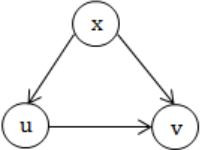Option A:
d[u] Option B:
d[u] Option C:
f[u] So, option D is True.
 Question 25

What is the largest integer m such that every simple connected graph with n vertices and n edges contains at least m different spanning trees?

 A 1 B 2 C 3 D n
Algorithms        Minimum-Spanning-Tree
Question 25 Explanation:
If graph is connected and contains n vertices and n edges means there is possibility of only one cycle.
→ If we create a different spanning tree by removing one edge at a time.
→ For cycle required 3 minimum edges. So there is at least 3 spanning trees in such a graph.
 Question 26

Consider n jobs J1, J2,......Jn such that job Ji has execution time ti and a non-negative integer weight wi. The weighted mean completion time of the jobs is defined to be, where Ti is the completion time of job Ji. Assuming that there is only one processor available, in what order must the jobs be executed in order to minimize the weighted mean completion time of the jobs?

 A Non-decreasing order of ti B Non-increasing order of wi C Non-increasing order of witi D None-increasing order of wi/ti
Operating-Systems       Process-Scheduling
Question 26 Explanation:
Like OS concept, execute job which have more completion time in 1 sec i.e., find wi/ti for every process then arrange it in the decreasing order. So, we get complete time is minimum always.
So answer is the non-increasing order of wi/ti.
 Question 27

The function f is defined as follows:

```int f (int n) {
if (n <= 1) return 1;
else if (n % 2  ==  0) return f(n/2);
else return f(3n - 1);
} ```
Assuming that arbitrarily large integers can be passed as a parameter to the function, consider the following statements.
(i) The function f terminates for finitely many different values of n ≥ 1.
(ii) The function f terminates for infinitely many different values of n ≥ 1.
(iii) The function f does not terminate for finitely many different values of n ≥ 1.
(iv) The function f does not terminate for infinitely many different values of n ≥ 1.
Which one of the following options is true of the above?

 A (i) and (iii) B (i) and (iv) C (ii) and (iii) D (ii) and (iv)
Programming       Programming
Question 27 Explanation:
The function can be terminated for all the values which can have factor of 2{(2-x)2 == 0}, so (i) is false and (ii) is true.
→ Let n=3, then it is terminated in 2nd iteration.
→ Let n=5, then sequence is 5→14→7→20→10 and it will repeat.
→ Any number with factor 5 and 2 leads to infinite recursion.
So, (iv) is True and (iii) is False.
 Question 28

Consider a hash function that distributes keys uniformly. The hash table size is 20. After hashing of how many keys will the probability that any new key hashed collides with an existing one exceed 0.5.

 A 5 B 6 C 7 D 10
Engineering-Mathematics       Probability
Question 28 Explanation:
Total spaces = 20, no. of entries = 1
Probability of collision for each entry = 1/20
After inserting X values then probability becomes 1/2
i.e., (1/20)X = 1/2
X = b
 Question 29

When searching for the key value 60 in a binary search tree, nodes containing the key values 10, 20, 40, 50, 70 80, 90 are traversed, not necessarily in the order given. How many different orders are possible in which these key values can occur on the search path from the root to the node containing the value 60?

 A 35 B 64 C 128 D 5040
Data-Structures       Binary-Trees
Question 29 Explanation:
To find 60 in BST then there are two possible set i.e., greater than 60 and smaller than 60.
Smaller values 90, 80 and 70 are visited in order
i.e., 7!/(4!3!) = 35
 Question 30

Suppose you are given an implementation of a queue of integers. The operations that can be performed on the queue are:
(i) isEmpty (Q) — returns true if the queue is empty, false otherwise.
(ii) delete (Q) — deletes the element at the front of the queue and returns its value.
(iii) insert (Q, i) — inserts the integer i at the rear of the queue.
Consider the following function:

```void f (queue Q) {
int i ;
if (!isEmpty(Q)) {
i = delete(Q);
f(Q);
insert(Q, i);
}
} ```
What operation is performed by the above function f?

 A Leaves the queue Q unchanged B Reverses the order of the elements in the queue Q C Deletes the element at the front of the queue Q and inserts it at the rear keeping the other elements in the same order D Empties the queue Q
Data-Structures       Queues
Question 30 Explanation:
As a recursive call, and removing from front while inserting from end, that means that element will be deleted at last and will be inserted 1st in the new queue. And like that it will continue till first call execute insert (Q, i) function. So the queue is in reverse order.
 Question 31

Consider the C program given below:

```#include
int main ()    {
int sum = 0, maxsum = 0,  i,  n = 6;
int a [] = {2, -2, -1, 3, 4, 2};
for (i = 0; i < n; i++)    {
if (i == 0 || a [i]  < 0  || a [i] < a [i - 1])  {
if (sum > maxsum) maxsum = sum;
sum = (a [i] > 0) ? a [i] : 0;
}
else sum += a [i];
}
if (sum > maxsum) maxsum = sum ;
printf ("%dn", maxsum);

} ```

What is the value printed out when this program is executed?

 A 9 B 8 C 7 D 6
Programming       C-Programming
Question 31 Explanation:
The algorithm is for finding the maximum sum of the monotonically increasing continuous sequence of positive numbers in the array. So, output will be 3+4 = 7.
 Question 32

Consider the following C program:

```   #include
#define EOF -1
void push (int); /* push the argument on the stack */
int pop  (void); /* pop the top of the stack */
void flagError ();

int main ()
{  int c, m, n, r;
while ((c = getchar ()) != EOF)
{     if  (isdigit (c) )
push (c);
else if ((c == '+') || (c == '*'))
{  m = pop ();
n = pop ();
r = (c == '+') ? n + m : n*m;
push (r);
}
else if (c != ' ')
flagError ();
}
printf("% c", pop ());
} ```
What is the output of the program for the following input?
`        5 2 * 3 3 2 + * + `

 A 15 B 25 C 30 D 150
Data-Structures       Stacks
Question 32 Explanation:
push 5
push 2
pop 2
pop 5
5 * 2 = 10
push 10
push 3
push 3
push 2
pop 2
pop 3
3 + 2 = 5
push 5
pop 5
pop 3
3 * 5 = 15
push 15
pop 15
pop 10
10 + 15 = 25
push 25
Finally, pop 25 and print it.
 Question 33

Consider the program below in a hypothetical language which allows global variable and a choice of call by reference or call by value methods of parameter passing.

```int i ;
program main ()
{
int j = 60;
i = 50;
call f(i,j);
print i, j;
}
procedure f(x,y)
{
i = 100;
x = 10;
y = y + i ;
} ```
Which one of the following options represents the correct output of the program for the two parameter passing mechanisms?

 A Call by value : i = 70, j = 10; Call by reference : i = 60, j = 70 B Call by value : i = 50, j = 60; Call by reference : i = 50, j = 70 C Call by value : i = 10, j = 70; Call by reference : i = 100, j = 60 D Call by value : i = 100, j = 60; Call by reference : i = 10, j = 70
Programming       Parameter-Passing
Question 33 Explanation:
Call by value:
'i' is a global variable. Then in main( ) a local variable 'j' as integer declared, i.e., j=60 and global variable 'i' is initialized to 50 by i=50.
Now procedure f called and values of 'i' and 'j' are passed to it, i.e., in f(i, j)→f(x, y).
Content of memory location of 'i' (here 50) is copied to memory location of x (which is different from i) and content of memory location of 'j' (here 60) is copied to memory location of y. Then in f(x, y) i=100 changes the global i to 100, x=10 changes the local x from 50 to 10 and y=y+i means y=60+100=160. Now, when return back to main, i & j will be 100 & 60 respectively.
Call by reference:
Now procedure f called and passed reference of i and j to it, i.e., in f(i,j)→f(x,y). x and y are pointing to same memory location of i and j respectively. So i=100 changes the global i to 100 and x=10 means as well as global i=10 (as the i being passed is the global variable and x and i share the same address).
y=y+i means y=60+10=70 and this changes the value of j also to as j and y have the same addresses. Now when return to main, i and j will be 10 and 70.
 Question 34

Consider the program below in a hypothetical programming language which allows global variables and a choice of static or dynamic scoping.

```int i ;
program main ()
{
i = 10;
call f();
}

procedure f()
{
int i = 20;
call g ();
}
procedure g ()
{
print i;
} ```
Let x be the value printed under static scoping and y be the value printed under dynamic scoping. Then, x and y are

 A x = 10, y = 10 B x = 20, y = 10 C x = 10, y = 20 D x = 20, y = 20
Programming       Variable Binding
Question 34 Explanation:
Since the value of x is based on static scoping, in the procedure g( ), print i will directly look into the global scope and find i=10 which was previously set by main( ) and since the value of y is based on dynamic scope, procedure g( ) will first look into the function which called it, i.e., procedure f( ) which has a local i=20, which will be taken and 20 will be printed.
 Question 35

Early binding refers to a binding performed at compile time and late binding refers to a binding performed at execution time. Consider the following statements:
i. Static scope facilitates w1 bindings.
ii. Dynamic scope requires w2 bindings.
iii. Early bindings w3 execution efficiency.
iv. Late bindings w4 execution efficiency.
The right choices of wl, w2, w3 and w4 (in that order) are

 A Early, late, decrease, increase B Late, early, increase, decrease C Late, early, decrease, increase D Early, late, increase, decrease
Programming       Variable Binding
Question 35 Explanation:
Static scoping can do early binding (during compile time). Early binding increases efficiency.
Dynamic scoping requires late binding (during execution time).
Late binding decreases efficiency as this binding needs to be done at run time.
 Question 36

The floating point unit of a processor using a design D takes 2t cycles compared to t cycles taken by the fixed point unit. There are two more design suggestions D1 and D2. D1 uses 30% more cycles for fixed point unit but 30% less cycles for floating point unit as compared to design D. D2 uses 40% less cycles for fixed point unit but 10% more cycles for floating point unit as compared to design D. For a given program which has 80% fixed point operations and 20% floating point operations, which of the following ordering reflects the relative performances of three designs? (Di > Dj denotes that Di is faster than Dj)

 A D1 > D > D2 B D2 > D > D1 C D > D2 > D1 D D > D1 > D2
Computer-Organization       Clock-Frequency
Question 36 Explanation:
T = 0.8 × time taken in fixed point + 0.2 × time taken in floating point
D = 0.8 × t + 0.2 × 2t = 1.2t
D1 = 0.8 × 1.3t + 0.2 × 0.7 × 2t = 1.32t
D2 = 0.8 × 0.6t + 0.2 × 1.1 × 2t = 0.92t
⇒ D2 > D > D1
 Question 37

Consider a Direct Mapped Cache with 8 cache blocks (numbered 0-7). If the memory block requests are in the following order

`3, 5, 2, 8, 0, 63, 9,16, 20, 17, 25, 18, 30, 24, 2, 63, 5, 82,17, 24.  `
Which of the following memory blocks will not be in the cache at the end of the sequence?

 A 3 B 18 C 20 D 30
Computer-Organization       Cache
Question 37 Explanation: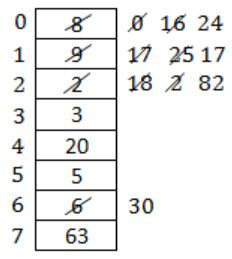So memory block 18 is not in the cache.
 Question 38

The following expression was to be realized using 2-input AND and OR gates. However, during the fabrication all 2-input AND gates were mistakenly substituted by 2-input NAND gates.

`(a.b).c + (a'.c).d + (b.c).d + a. d `
What is the function finally realized?

 A 1 B a’ + b’ + c’ + d’ C a’ + b + c’ + d’ D a’ + b’ + c + d’
Digital-Logic-Design       Boolean-Functions
Question 38 Explanation:
(a⋅b)⋅c + (a'⋅c)⋅d + (b⋅c)⋅d + a⋅d
= ((ab)'c)' + ((a'c)'d)' + ((bc)'d)' + (ad)'
= ab + c' + a'c + d' + bc + d' + a' + d'
= ab + c' + a'c + bc + a' + d'
= ab + c' + bc + a' + d'
= b + c' + bc + a' + d'
= a' + b + c' + d'
 Question 39

Data forwarding techniques can be used to speed up the operation in presence of data dependencies. Consider the following replacements of LHS with RHS.

```(i) R1→Loc, Loc→R2 ≡ R1→R2, R1→Loc
(ii) R1→Loc, Loc→R2 ≡ R1→R2
(iii) R1→Loc, R2→Loc ≡ R1→Loc
(iv) R1→Loc, R2→Loc ≡ R2→Loc ```
In which of the following options, will the result of executing the RHS be the same as executing the LHS irrespective of the instructions that follow?

 A (i) and (iii) B (i) and (iv) C (ii) and (iii) D (ii) and (iv) E Only (i)
Computer-Organization       Data-Dependencies
Question 39 Explanation:
(i) Is true. Both Loc and R2 are getting the value of R1 in LHS and RHS.
(ii) Is false. Because R2 gets the correct data in both LHS and RHS, but Loc is not updated in RHS.
(iii) Is false. Because R2 is writing last in LHS, but not in RHS.
(iv) Is true. The first write to Loc in LHS is useless as it is overwritten by the next write.
 Question 40

What is the final value stored in the linear feedback shift register if the input is 101101?A 0110 B 1011 C 1101 D 1111
Digital-Logic-Design       Shift-register
Question 40 Explanation: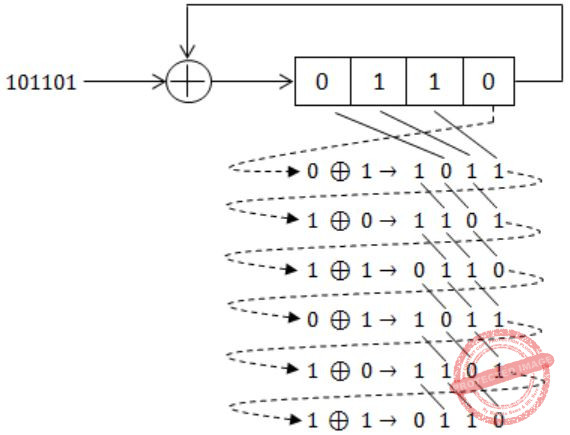Question 41

Following table indicates the latencies of operations between the instruction producing the result and instruction using the result.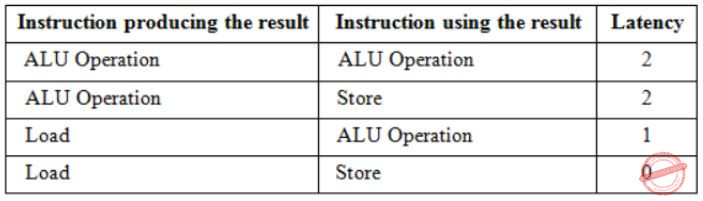Consider the following code segment.

```Load R1,Loc1;	 Load R1 from memory location Loc1
Load R2,Loc2;	 Load R2 from memory location Loc2
Add R1,R2,R1;	 Add R1 and R2 and save result in R1
Dec R2;	         Decrement R2
Dec R1;	         Decrement R1
Mpy R1,R2,R3;	 Multiply R1 and R2 and save result in R3
Store R3,Loc3;   Store R3 in memory location Loc3```
What is the number of cycles needed to execute the above code segment assuming each instruction takes one cycle to execute?

 A 7 B 10 C 13 D 14
Computer-Organization       Machine-Instructions
Question 41 Explanation:
In the given question, there are 7 instructions each of which takes 1 clock cycle to complete.
If an instruction is in execution phase then any other instructions cannot be in the execution phase. So, atleast 7 clock cycles will be taken.
Now, it is given that between two instructions latency or delay should be there based on their operation.
[Image_Link] 1) Load R1, Loc1;
Takes 1 clock cycle, simply loading R1 on Loc1.
2) Load R2, Loc2;
Takes 1 clock cycle.
3) Add R1, R2, R3;
Hence, this instruction is using the result of R1 and R2, i.e., result of Instruction 1 and Instruction 2. As Instruction 1 is load operation and Instruction 3 is ALU operation. So, there should be a delay of 1 clock cycle between Instruction 1 and Instruction 3, which is already there due to Instruction 2.
As Instruction 2 is load operation and Instruction 3 is ALU operation. So, there should be a delay of 1 clock cycle between Instruction 2 and Instruction 3.
4) Dec R2;
This instruction is dependent on Instruction 2 and there should be delay of one clock cycle between Instruction 2 and Instruction 4. As Instruction 2 is load and Instruction 4 is ALU, which is already there due to Instruction 2.
5) Dec R1;
This instruction is dependent on Instruction 3.
As Instruction 3 is ALU and Instruction 5 is also ALU, so a delay of 2 clock cycles should be there between them of which 1 clock cycle is already there due to Instruction 4. So, one clock delay between Instruction 4 and Instruction 5.
6) MPY R1, R2, R3;
This instruction uses the result of Instruction 5, as both Instruction 5 and Instruction 6 are ALU. So, there should be delay of 2 clock cycles.
7) Store R3, Loc3;
This instruction is dependent on Instruction 6 which is ALU and Instruction 7 is stored. So, there should be a delay of 2 clock cycles between them.
 Question 42
(C012.25)H – (10111001110.101)B =

 A (135103.412)O B (564411.412)O C (564411.205)O D (135103.205)O
Digital-Logic-Design       Number-Systems
Question 42 Explanation:
(C012.25)H – (10111001110.101)B
= 1100000000010010.00100101 - 0000010111001110.10100000
= 1011101001000011.10000101
= 1011101000011.100001010
= (135103.412)O
 Question 43

An error correcting code has the following code words:

`00000000, 00001111, 01010101, 10101010, 11110000. `
What is the maximum number of bit errors that can be corrected?

 A 0 B 1 C 2 D 3
Computer-Networks       Error-Correction
Question 43 Explanation:
For correction:
The no. of bits that can be corrected isQuestion 44

A hard disk system has the following parameters:
Number of tracks = 500
Number of sectors/track = 100
Number of bytes /sector = 500
Time taken by the head to move from one track to adjacent track = 1 ms
Rotation speed = 600 rpm.
What is the average time taken for transferring 250 bytes from the disk ?

 A 300.5 ms B 255.5 ms C 255.0 ms D 300.0 ms
Computer-Organization       Secondary-Storage
Question 44 Explanation:
Avg. time to transfer = Avg. seek time + Avg. rotational delay + Data transfer time
For Avg. seek time:
Given that, time to move between successive tracks is 1ms.
Time to move from track1 to track1 = 0ms
Time to move from track1 to track2 = 1ms
Time to move from track1 to track3 = 2ms
⋮ Time to move from track1 to track500 = 499ms
∴ Avg. seek time = 0+1+2+...+499/500 = 249.5ms
Avg. rotational delay:
600 rotations in 60sec.
One rotation takes 60/600 s = 100ms ∴ Avg. rotational delay = 100/2 = 50ms
Data transfer time:
In one rotation, we can read data on one complete track
= 100 × 500 = 50,000B data is read in one complete rotation
One complete rotation takes 100ms.
100ms → 50,000B
250B → 100/50000 × 250 = 0.5ms
∴ Avg. time to transfer = 249.5 × 50 + 0.5 = 300ms
 Question 45

The line T in the following figure is permanently connected to the ground.Which of the following inputs (X1 X2 X3 X4) will detect the fault?

 A 0000 B 0111 C 1111 D None of these
Digital-Logic-Design       Circuits-Output
Question 45 Explanation: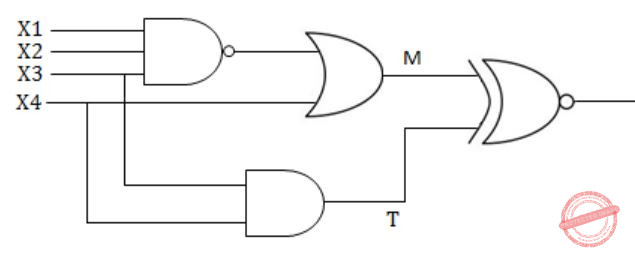Since the problem is in the link T which is connected as input to NOR gate. So to check link T we have to make the output dependent on T by deactivating link M. So to deactivate link M, the output at M should be 0, as link M is input to NOR gate. So, to output at M as 0,
X1 = 1
X2 = 1
X3 = 1
X4 = 0
∴ None of the given option is correct.
 Question 46

The two grammars given below generate a language over the alphabet {x, y, z}

```G1: S → x|z|xS|zS|yB
B → y|z|yB|zB
G2: S → y|z|yS|zS|xB
B → y|yS ```

Which one of the following choices describes the properties satisfied by the strings in these languages?

 A G1 : No y appears before any x G2 : Every x is followed by at least one y B G1 : No y appears before any x G2 : No x appears before any y C G1 : No y appears after any x G2 : Every x is followed by at least one y D G1 : No y appears after any x G2 : Every y is followed by at least one x
Theory-of-Computation        Grammar
Question 46 Explanation:
Regular expression for G1:
(x + z)* + (x + z)* y(y + z)*
Regular expression for G2:
(y + z + xy)*
 Question 47

Consider the following DFA in which s0 is the start state and s1, s3 are the final states.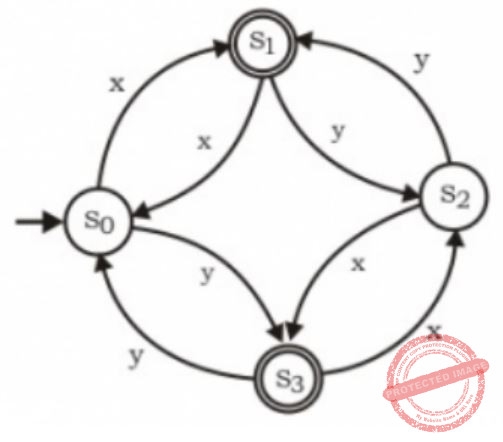What language does this DFA recognize ?

 A All strings of x and y B All strings of x and y which have either even number of x and even number of y or odd number or x and odd number of y C All strings of x and y which have equal number of x and y D All strings of x and y with either even number of x and odd number of y or odd number of x and even number of y
Theory-of-Computation       Finite-Automata
Question 47 Explanation:
Just simulate the running of the DFA on all options - A, B and C are false and D is true.
 Question 48

Consider the grammar given below

```S → x B | y A
A → x | x S | y A A
B → y | y S | y B B ```

Consider the following strings.

```(i) xxyyx
(ii) xxyyxy
(iii) xyxy
(iv) yxxy
(v) yxx
(vi) xyx ```

Which of the above strings are generated by the grammar ?

 A (i), (ii), and (iii) B (ii), (v), and (vi) C (ii), (iii), and (iv) D (i), (iii), and (iv)
Theory-of-Computation       Grammar
Question 48 Explanation:
(ii) xxyyxy
S → xB
S → xxBB
S → xxyB
S → xxyyS
S → xxyyxB
S → xxyyxy
(iii) xyxy
S → xB
S → xyS
S → xyxB
S → xyxy
(iv) yxxy
S → yA
S → yxS
S → yxxB
S → yxxy
 Question 49

Consider the following grammars. Names representing terminals have been specified in capital letters.

```G1: stmnt → WHILE (expr) stmnt
stmnt → OTHER
expr → ID
G2: WHILE (expr) stmnt
stmnt → OTHER
expr → expr + expr
expr → expr * expr
expr → ID   ```

Which one of the following statements is true?

 A G1 is context-free but not regular and G2 is regular B G2 is context-free but not regular and G1 is regular C Both G1 and G2 are regular D Both G1 and G2 are context-free but neither of them is regular
Theory-of-Computation       Identify-Class-Language
Question 49 Explanation:
Regular grammar is either right linear or left linear. A left linear grammar is one in which there is atmost 1 non-termial on the right side of any production, and it appears at the left most position.
Similarly, in right linear grammar, non-terminal appears at the right most position.
Here we can write a right linear grammar for G1 as
S → w(E
E → id)S
S → o
(w-while, o-other)
So, L(G1) is regular.
Now for G2 also we can write a right linear grammar:
S → w(E
E → id)S
E → id+E
E → id*E
S → o
making its language regular.
So, both G1 and G2 have an equivalent regular grammar. But given in the question both these grammars are neither right linear nor left linear and hence not a regular grammar. So, (D) must be the answer.
 Question 50

Consider the following finite automata P and Q over the alphabet {a, b, c}. The start states are indicated by a double arrow and final states are indicated by a double circle. Let the languages recognized by them be denoted by L(P) and L(Q) respectively.The automation which recognizes the language L(P) ∩ L(Q) is:

 AB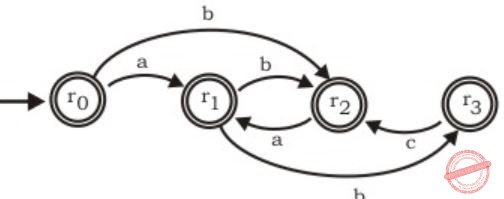CDTheory-of-Computation       Finite-Automata
Question 50 Explanation:
String 'aca' is accepted by both FA, P and Q. And FA in option (A) also accepts string 'aa' and none of the other option FA accepts 'aa'. Hence option (A) is the answer.
 Question 51

The following table shoes the time between failures for a software system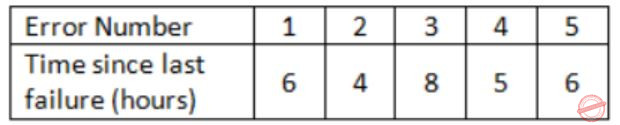The reliability of the system for one hour of operation assuming an exponential model is

 A 0.45 B 0.63 C 0.84 D 0.95
Question 51 Explanation:
MIBF = ∑(Start of downtime - Start of uptime)/No. of failures
MIBF = (6+4+8+5+6)/5 = 29/5
The probability or reliability that the product will work for a defined period of time without failure is given by
R(T) = exp(-T/MTBF); T = 1 hour
R(1) = e(-1/(29/5)) = e(-5/29) = 0.84
 Question 52

Given the following algorithm for sorting an array X of N numbers:

```SUBROUTINE SORT(X,N)
IF(N < 2)
RETURN
FOR(i=2 TO N INCREMENT BY 1)
FOR(j=1 TO i INCREMENT BY 1)
IF (X[i] > X[j])
CONTINUE
TEMP X[i]
X[i] = X[j]
X[j] = TEMP
END FOR
END FOR
END SUBROUTINE ```

A good approximation of Halstead's estimated program length is

 A 20 B 50 C 80 D 110
 Question 53

In the simplified flowchart given below, the shaded boxes represent code that is executed during a test case.The Branch coverage is

 A 3/4 B 2/3 C 1/2 D 3/8
Question 53 Explanation:
Note: Out of syllabus.
 Question 54

Consider the CPM activity chart where an arc connecting two milestones is labeled with a task identifier and the time taken in days. For example in order to go from A to B, task T1 takes 180 days. A dashed line depicts an additional dependency that is equivalent to a zero time task.The set of activities that lie on the critical path are

 A T1, T2, T8, T10 B T1, T3, T8, T10 C T1, T2, T3, T4, T5, T6, T7, T8, T9, T10 D T1, T4, T5, T7, T8, T10
Question 54 Explanation:
Note: Out of syllabus.
 Question 55

Consider the following pseudo-code:

``` IF ((A > B) AND (C > D)) THEN
A = A + 1
B = B + 1
ENDIF
```

The cyclomatic complexity of the pseudo-code is

 A 2 B 3 C 4 D 5
Question 55 Explanation:
Note: Out of syllabus.
 Question 56

Synchronization in the classical readers and writers problem can be achieved through use of semaphores. In the following incomplete code for readers-writers problem, two binary semaphores mutex and wrt are used to obtain synchronization

```wait (wrt)
writing is performed
signal (wrt)
wait (mutex)
readcount = readcount + 1
if readcount = 1 then S1
S2
reading is performed
S3
readcount = readcount - 1
if readcount = 0 then S4
signal (mutex) ```

The values of S1, S2, S3, S4, (in that order) are

 A signal (mutex), wait (wrt), signal (wrt), wait (mutex) B signal (wrt), signal (mutex), wait (mutex), wait (wrt) C wait (wrt), signal (mutex), wait (mutex), signal (wrt) D signal (mutex), wait (mutex), signal (mutex), wait (mutex)
Operating-Systems        Process-Synchronization
Question 56 Explanation:
S1: If readcount is 1, i.e., some reader is reading, DOWN on wrt so that no writer can write.
S2: After readcount has been updated, UP on mutex.
S3: DOWN on mutex to update readcount.
S4: If readcount is zero, i.e., no reader is reading, UP on wrt to allow some writer to write.
 Question 57

In a multi-user operating system on an average, 20 requests are made to use a particular resource per hour. The arrival of requests follows a Poisson distribution. The probability that either one, three or five requests are made in 45 minutes is given by :

 A 6.9 × 106 × e-20 B 1.02 × 106 × e-20 C 6.9 × 103 × e-20 D 1.02 × 103 × e-20
Engineering-Mathematics       Probability
Question 57 Explanation:
q0 request in 1 hour. So we can expect 15 requests in 45 minutes.
So, λ=15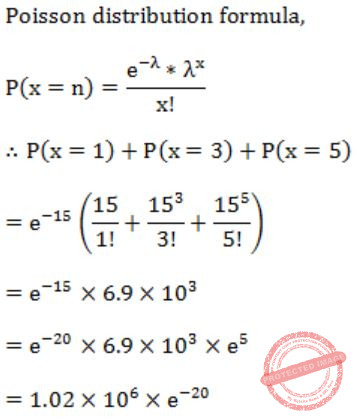Question 58

A demand paging system takes 100 time units to service a page fault and 300 time units to replace a dirty page. Memory access time is 1 time unit. The probability of a page fault is p. In case of a page fault, the probability of page being dirty is also p. It is observed that the average access time is 3 time units. Then the value of p is

 A 0.194 B 0.233 C 0.514 D 0.981 E 0.0194
Operating-Systems       Virtual Memory
Question 58 Explanation:
Given page fault service time = 100
(if the page is not dirty)
Page fault service time = 300
(if there is dirty page)
Probability of page fault = P
Probability pf page being dirty = P
So, total page fault service time
= P(300) + (1 - P)100
= 300P + 100 - 100P
= 300P + 100
Now given,
Effective average memory access time = 3
So,
3 = m + P × total page fault service time
= 1 + P(200P + 100)
= 1+ 200P2 + 100P
⇒ 200P2 + 100P - 2 = 0
⇒ P = 0.0194
 Question 59

The contents of the text file t1.txt containing four lines are as follows:

```a1 b1
a2 b2
a3 b2
a4 b1 ```

The contents of the text file t2.txt containing five lines are as follows:

```a1 c1
a2 c2
a3 c3
a4 c3
a5 c4 ```

Consider the following Bourne shell script:

```awk - F''' {Print S1, S2} ' t1.txt |
while read a b ; do
awk -v aV = Sa - v bV = Sb - F''
'aV = = S1 (print aV, bV, S2 )'t2.txt
done ```

Which one of the following strings will NOT be present in the output generated when the above script in run? (Note that the given strings may be substrings of a printed line.)

 A "b1 c1" B "b2 c3" C "b1 c2" D "b1 c3"
Question 59 Explanation:
Note: Out of syllabus.
 Question 60

For the network given in the figure below, the routing tables of the four nodes A, E, D and G are shown. Suppose that F has estimated its delay to its neighbors, A, E, D and G as 8, 10, 12 and 6 msecs respectively and updates its routing table using distance vector routing technique.AB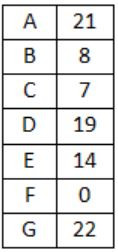CDComputer-Networks       Routing
Question 60 Explanation:
Distance from F to F is 0 which eliminates option (C).
Using distance vector routing protocol, F→D→B yeilds distance as 20 which eliminates option (B) and (D).
 Question 61

In the waveform (a) given below, a bit stream is encoded by Manchester encoding scheme. The same bit stream is encoded in a different coding scheme in wave form (b). The bit stream and the coding scheme areA 1000010111 and Differential Manchester respectively B 0111101000 and Differential Manchester respectively C 1000010111 and Integral Manchester respectively D 0111101000 and Integral Manchester respectively
Computer-Networks       Manchester-Encoding
Question 61 Explanation:
Both (A) & (B) is correct, it is just the convention which determine the correct answer.
As per IEEE (B) is correct.
As per GE Thomas (A) is correct.
As we usually follow IEEE thus (B) is correct.
 Question 62

Let us consider a statistical time division multiplexing of packets. The number of sources is 10. In a time unit, a source transmits a packet of 1000 bits. The number of sources sending data for the first 20 time units is 6, 9, 3, 7, 2, 2, 2, 3, 4, 6, 1, 10, 7, 5, 8, 3, 6, 2, 9, 5 respectively. The output capacity of multiplexer is 5000 bits per time unit. Then the average number of backlogged of packets per time unit during the given period is

 A 5 B 4.45 C 3.45 D 0
Computer-Networks       Access-Control-Methods
Question 62 Explanation:
The capacity of multiplexer is 5000 bits per time unit. This means there are 5 packets per unit time since each source transmits a packet of 1000 bits in a unit time.
If the no. of packets transmitted is larger than 5 then the extra packets are backlogged. This means gets added to the next number and further backlog is calculated.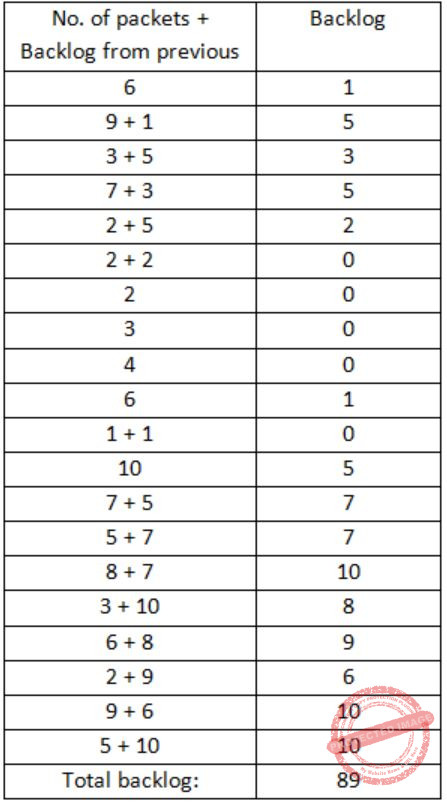Average no. of backlogged packets = 89/20 = 4.45
 Question 63

A group of 15 routers are interconnected in a centralized complete binary tree with a router at each tree node. Router j communicates with router j by sending a message to the root of the tree. The root then sends the message back down to router j. The mean number of hops per message, assuming all possible router pairs are equally likely is

 A 3 B 4.26 C 4.53 D 5.26
Computer-Networks       Routing
Question 63 Explanation:
Similarly, average hops when message comes down
= (3×8) + (2×4) + (1×2) + (0×1)/15
= 2.267
So, Total hops = 2×2.267 = 4.53
 Question 64

A broadcast channel has 10 nodes and total capacity of 10 Mbps. It uses polling for medium access. Once a node finishes transmission, there is a polling delay of 80 μs to poll the next node. Whenever a node is polled, it is allowed to transmit a maximum of 1000 bytes. The maximum throughput of the broadcast channel is

 A 1 Mbps B 100/11 Mbps C 10 Mbps D 100 Mbps
Computer-Networks       Access-Control-Methods
Question 64 Explanation:
Efficiency = Transmission time(Tt)/Transmission time+Polling delay
(Tt) = 1000bytes/10×106bits/sec = 800μs
Polling delay = 80 μs
Efficiency = 800/800+80 = 800/880 = 10/11
Maximum throughput is
= 10/11 × 10 Mbps
= 100/11 Mbps
 Question 65

Consider a selection of the form σA≤100(r), where r is a relation with 1000 tuples. Assume that the attribute values for A among the tuples are uniformly distributed in the interval [0, 500]. Which one of the following options is the best estimate of the number of tuples returned by the given selection query ?

 A 50 B 100 C 150 D 200
Database-Management-System       Relational-Algebra
Question 65 Explanation:
σA≤100(r), r has 1000 tuples.
Values for A among the tuples are uniformly distributed in the interval [0, 500]. This can be split to 5 mutually exclusive and exhaustive intervals of same width of 100 ([0-100], [101-200], [201-300], [301-400], [401-500], 0 makes the first interval larger - this must be type in this question) and we can assume all of them have same number of values due to uniform distribution. So no. of tuples with A value in first interval should be,
Total no. of tuples/5 = 1000/5 = 200
 Question 66

Consider the following two transactions: T1 and T2.

```T1: read(A);
read(B);
if A=0 then B ← B+1;
write(B);
T2: read(B);
read(A);
if B≠0 then A ← A-1;
write(A);
Which of the following schemes, using shared and exclusive locks, satisfy the requirements for strict two phase locking for the above transactions?
```
 ABC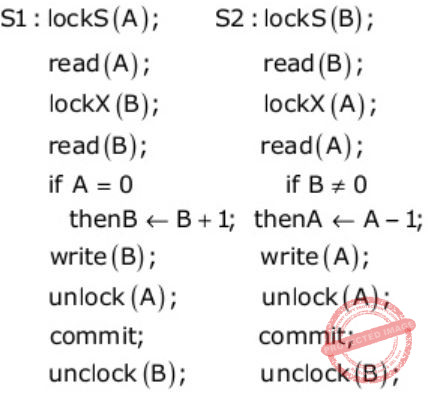DDatabase-Management-System       Transactions
Question 66 Explanation:
For strict 2PL the requirements are,
i) Exclusive locks should be released after the commit.
ii) No locking can be done after the first unlock.
(A) Wrong because to write x you need Exclusive Lock.
(B) Wrong because violating the 1st requirement.
(C) Correct.
(D) Wrong because violating the 1st requirement.
 Question 67

Consider the following implications relating to functional and multivalued dependencies given below, which may or may not be correct.
(i) If A ↠ B and A ↠ C then A → BC
(ii) If A → B and A → C then A ↠ BC
(iii) If A ↠ BC and A → B then A → C
(iv) If A → BC and A → B then A ↠ C
Exactly how many of the above implications are valid?

 A 0 B 1 C 2 D 3
Database-Management-System       Multi-valued-Dependencies
Question 67 Explanation:
The rule is
if A→B then A→→B holds but reverse is not true.
So,
(i) False.
(ii) True.
(iii) False.
(iv) True.
 Question 68

Consider the following relation schemas:
b-Schema = (b-name, b-city, assets)
a-Schema = (a-num, b-name, bal)
d-Schema = (c-name, a-number)

Let branch, account and depositor be respectively instances of the above schemas. Assume that account and depositor relations are much bigger than the branch relation.

Consider the following query:

` Пc-name (σb-city = "Agra" ⋀ bal < 0 (branch ⋈ (account ⋈ depositor)  `

Which one of the following queries is the most efficient version of the above query?

 A Пc-name (σbal < 0 (σb-city = “Agra” branch ⋈ account) ⋈ depositor) B Пc-name (σb-city = “Agra”branch ⋈ (σbal < 0 account ⋈ depositor)) C Пc-name (σb-city = “Agra” branch ⋈ σb-city = “Agra” ⋀ bal < 0 account) ⋈ depositor) D Пc-name (σb-city = “Agra” ⋀ bal < 0 account ⋈ depositor))
Database-Management-System       Relational-Algebra
Question 68 Explanation:
Answer should be (A) and not (B), because we are doing a join between two massive tables whereas in (A) we are doing join between relatively smaller table and larger one and the output that this inner table gives (which is smaller in comparison to join that we are doing in (B)) is used for join with depositor table with the selection condition.
Options (C) and (D) are invalid as there is no b-city column in a-schema.
 Question 69

Consider the following clauses:
(i) Not inherently suitable for client authentication.
(ii) Not a state sensitive protocol.
(iii) Must be operated with more than one server.
(iv) Suitable for structured message organization.
(v) May need two ports on the serve side for proper operation.
The option that has the maximum number of correct matches is

 A IMAP-(i), FTP-(ii), HTTP-(iii), DNS-(iv), POP3-(v) B FTP-(i), POP3-(ii), SMTP-(iii), HTTP-(iv), IMAP-(v) C POP3-(i), SMTP-(ii), DNS-(iii), IMAP-(iv), HTTP-(v) D SMTP-(i), HTTP-(ii), IMAP-(iii), DNS-(iv), FTP-(v)
Computer-Networks       Network-Protocols
Question 69 Explanation:
(ii) HTTP as it does not depend on state of device or operating system.
(v) FTP needs two ports, 20 for data and 21 for control.
Hence, only (D) matches.
 Question 70

Your are given the following four bytes :

` 10100011         00110111         11101001         10101011  `
Which of the following are substrings of the base 64 encoding of the above four bytes?

 A zdp B fpq C qwA D oze
Computer-Networks       Network-Security
Question 70 Explanation:
You are given the following four bytes:
10100011 00110111 11101001 10101011
So, in total we have 32 bits. And for base 64 we need 6 digits of binary no. to represent one digit of base 64 no.
So lets padd 4 bits on RHS, so that total digits will become 36 and we can separate then as group of 6 digits each.Now, the longest substring will be from checking option is 'fpq'.
 Question 71

Consider the regular expression

` R = (a + b)* (aa + bb) (a + b)* `
Which of the following non-deterministic finite automata recognizes the language defined by the regular expression R? Edges labeled λ denote transitions on the empty string.

 ABC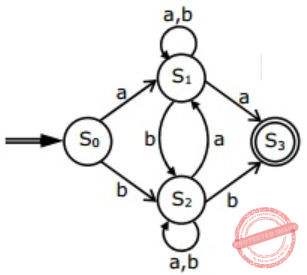DTheory-of-Computation       Regular-Expressions
Question 71 Explanation:
Every string of given regular expression must contain the substring aa or bb.
But option (B), (C), (D) accepts aba, which do not contain aa or bb as substring.
Hence, (A) is correct.
 Question 72

Consider the regular expression

` R = (a + b)* (aa + bb) (a + b)* `
Which deterministic finite automaton accepts the language represented by the regular expression R ?

 A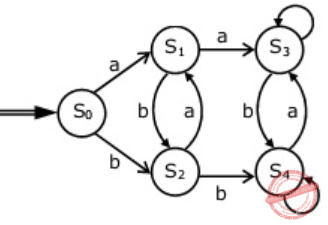B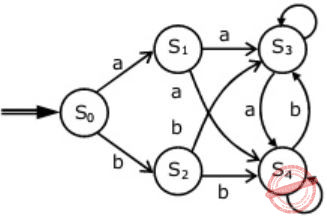CDTheory-of-Computation       Regular-Expressions
Question 72 Explanation:
(B) It accepts 'ab' which is not in the language.
(C) It is not accepting 'abb' which is in language.
(D) It is not accepting 'aa' and 'bb' which is in language.
 Question 73

Consider the regular expression

` R = (a + b)* (aa + bb) (a + b)* `
Which one of the regular expressions given below defines the same language as defined by the regular expression R?

 A (a(ba)* + b(ab)*)(a + b)+ B (a(ba)* + b(ab)*)*(a + b)* C (a(ba)* (a + bb) + b(ab)*(b + aa))(a + b)* D (a(ba)* (a + bb) + b(ab)*(b + aa))(a + b)+
Theory-of-Computation       Regular-Expressions
Question 73 Explanation:
R = (a+b)* (aa+bb) (a+b)*
Having NFA: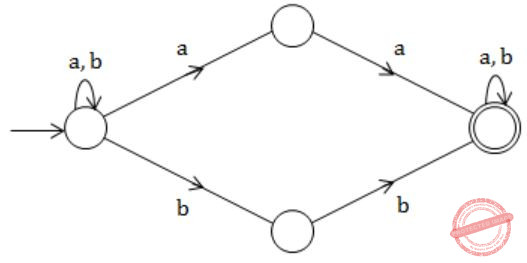Equivalent DFA:Question 74

Consider a token ring topology with N stations (numbered 1 to N) running token ring protocol where the stations are equally spaced. When a station gets the token it is allowed to send one frame of fixed size. Ring latency is tp, while the transmission time of a frame is tt. All other latencies can be neglected.
The maximum utilization of the token ring when tt = 3 ms, tp = 5 ms, N = 10 is

 A 0.545 B 0.6 C 0.857 D 0.961
Computer-Networks       Token-Ring-Topology
Question 74 Explanation:
Note: Out of syllabus.
 Question 75

Consider a token ring topology with N stations (numbered 1 to N) running token ring protocol where the stations are equally spaced. When a station gets the token it is allowed to send one frame of fixed size. Ring latency is tp, while the transmission time of a frame is tt. All other latencies can be neglected.
The maximum utilization of the token ring when tt = 5 ms, tp = 3 ms, N = 15 is:

 A 0.545 B 0.655 C 0.9375 D 0.961
Computer-Networks       Token Ring Topology
Question 75 Explanation:
Note: Out of syllabus.
 Question 76

Consider the sequence <xn>, n>= 0 defined by the recurrence relation xn + 1 = c . xn2- 2, where c > 0.
Suppose there exists a non-empty, open interval (a, b) such that for all x0 satisfying a < x0 < b, the sequence converges to a limit. The sequence converges to the value?

 ABC 2 DEngineering-Mathematics       Combinatorics
Question 76 Explanation:
Let c=1, x0=1
Then,
x1 = c ⋅ (x0)2 - 2 = 1 ⋅ (1)2 - 2 = -1
x2 = c ⋅ (x1)2 - 2 = 1 ⋅ (-1)2 - 2 = -1
So, the value converges to -1, which is equal toExactly, only (B) is answer. As all the term of x converges to -1.
 Question 77

Consider the sequence <xn>, n>= 0 defined by the recurrence relation xn + 1 = c. xn2- 2, where c > 0.
For which of the following values of c, does there exist a non-empty open interval (a,b) such that the sequence xn, converges for all x0 satisfying a < x0

(i) 0.25
(ii) 0.35
(iii) 0.45
(iv) 0.5
 A (i) Only B (i) and (ii) only C (i), (ii) and (iii) only D (i), (ii), (iii) and (iv)
Engineering-Mathematics       Combinatorics
Question 77 Explanation:
For the series to converge the limit: 'n' tends to infinity of (xn+1 / xn) should be <1.
From the recurrence we should have c(xn)2 - xn - 2 < 0
For all the above values of c we have above equation as negative.
 Question 78

Consider the following expression

` ad' + (ac)' + bc'd `
Which of the following Karnaugh Maps correctly represents the expression?

 ABCDDigital-Logic-Design       K-Map
Question 78 Explanation:
Just draw the K-map from the given expression,
ad' + a'c' + bc'd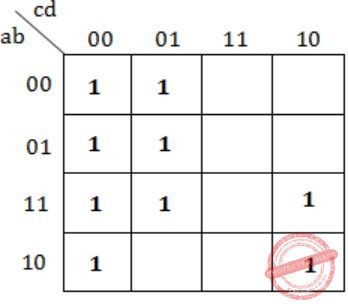Hence, option (A) matches.
 Question 79

Consider the following expression

` ad' + (ac)' + bc'd `
Which of the following expressions does not correspond to the Karnaugh Map obtained for the above expression?

 A c’d’+ ad’ + abc’ + (ac)’d B (ac)’ + c’d’ + ad’ + abc’d C (ac)’ + ad’ + abc’ + c’d D b’c’d’ + acd’ + (ac)’ + abc’
Digital-Logic-Design       K-Map
Question 79 Explanation:
Let's check for option (C):
a'c' + ad' + abc' + c'd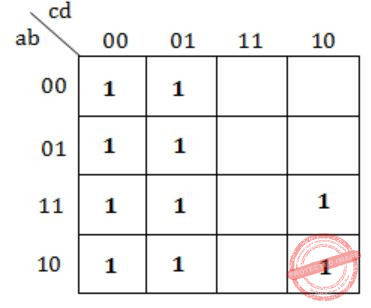Not equivalent to the K-map, we get in previous question.
 Question 80

Let P1, P2,..... , Pn be n points in the xy-plane such that no three of them are collinear. For every pair of points Pi and Pj, let Lij be the line passing through them. Let Lab be the line with the steepest gradient amongst all n(n -1)/2 lines.
Which one of the following properties should necessarily be satisfied?

 A Pa and Pb are adjacent to each other with respect to their x-coordinate B Either Pa or Pb has the largest or the smallest y-coordinate among all the points C The difference between x-coordinates Pa and Pb is minimum D None of the above
Engineering-Mathematics       Lines-and-Curves
Question 80 Explanation:
Draw the line from the first to third point, and look at whether the middle point is above, at or below it. If the middle point is on the line, all slopes are equal. If the middle point is above the line, then the slope from the first to the second point is the highest. If the middle point is below the line, then the slope from the middle point to the second is highest. In any case, you can find a greater slope between two adjacent points.
 Question 81

Let P1,P2,…,Pn be n points in the xy-plane such that no three of them are collinear. For every pair of points Pi and Pj, let Lij be the line passing through them. Let Lab be the line with the steepest gradient among all n(n−1)/2 lines.
The time complexity of the best algorithm for finding Pa and Pb is

 A Θ(n) B Θ(nlogn) C Θ(nlog2n) D Θ(n2)
Engineering-Mathematics       Lines-and-Curves
Question 81 Explanation: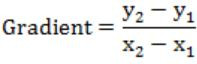For gradient to be maximum x2-x1 should be minimum. So, sort the points (in Θ(n logn) time) according to n coordinate and find the minimum difference between them (in Θ(n) time).
∴ Complexity = Θ(n logn + n) = Θ(n logn)
 Question 82

The head of a hard disk serves requests following the shortest seek time first (SSTF) policy. The head is initially positioned at truck number 180.
Which of the request sets will cause the head to change its direction after servicing every request assuming that the head does not change direction if there is a tie in SSTF and all the requests arrive before the servicing starts?

 A 11, 139, 170, 178, 181, 184, 201, 265 B 10, 138, 170, 178, 181, 185, 201, 265 C 10, 139, 169, 178, 181, 184, 201, 265 D 10, 138, 170, 178, 181, 185, 200, 265
Operating-Systems       Disk-Scheduling
Question 82 Explanation:Hence, correct option is (B).
 Question 83

The head of a hard disk serves requests following the shortest seek time first (SSTF) policy. The head is initially positioned at track number 180.
What is the maximum cardinality of the request set, so that the head changes its direction after servicing every request if the total number of tracks are 2048 and the head can start from any track?

 A 9 B 10 C 11 D 12
Operating-Systems       Disk-Scheduling
Question 83 Explanation:
We need two conditions to satisfy:
1) The alternating direction with SSTF policy.
2) Maximize the no. of requests.
The first condition can be satisfied by not having two requests in the equal distance from the current location. As shown below, we must not have request located in the circle marked positions.
Now to maximize the no. of request we need the requests to be located as compact as possible, which can be done by just placing the request in the next position after the circle marked position in particular direction (the direction in which the head need to move).
Now to satisfy the 1st criteria:Seek length sequence for maximum cardinality and alternating head movements:
→ 1, 3, 7, 15, ...
→ or, 21-1, 22-1, 23-1, 24-1, ...
→ We have 2048 tracks so, maximum swing (seek length) can be 2047.
→ Which corresponds to a seek length of 211-1 in the 11th service.
 Question 84

Consider the B+ tree in the adjoining figure, where each node has atmost two keys and three links.Keys K15 and then K25 are inserted into this tree in that order. Exactly how many of the following nodes (disregarding the links) will be present in the tree after the two insertions?A 1 B 2 C 3 D 4
Database-Management-System       B+-Trees
Question 84 Explanation:
It is B+ Tree.
After inserting K15 we get,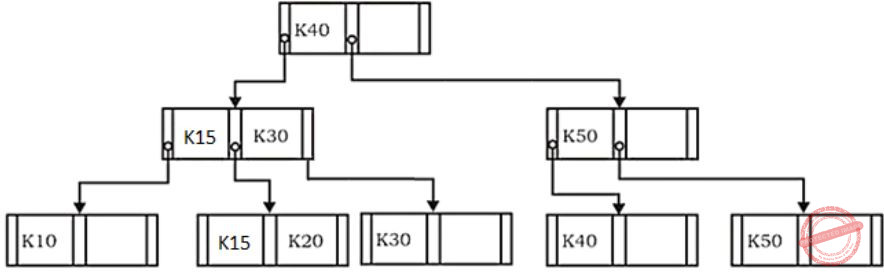Now, we insert K25, which givesSo, we see in the final tree only (K20, K25) is present. Hence, 1 (Answer).
 Question 85

Consider the B+ tree in the adjoining figure, where each node has atmost two keys and three links.Now the key K50 is deleted from the 8 tree resulting after the two insertions made earlier. Consider the following statements about the B tree resulting after this deletion.
(i) The height of the tree remains the same.
(ii) The node (disregarding the links) is present in the tree.
(iii) The root node remains unchanged (disregarding the links).
Which one of the following options is true?

 A Statements (i) and (ii) are true B Statements (ii) and (iii) are true C Statements (iii) and (i) are true D All the statements are false
Database-Management-System       B+-Trees
Question 85 Explanation:
The B+ tree in previous question we get was,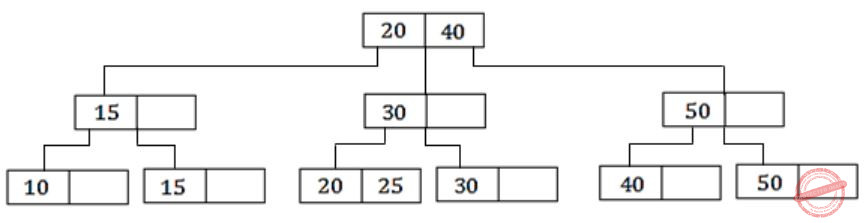Now after deleting 50 we get B+ tree as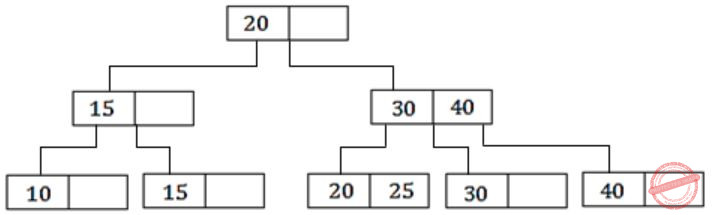Hence, correct statement is only (i) & (ii).
There are 85 questions to complete.
PHP Code Snippets Powered By : XYZScripts.com
error: Content is protected !!